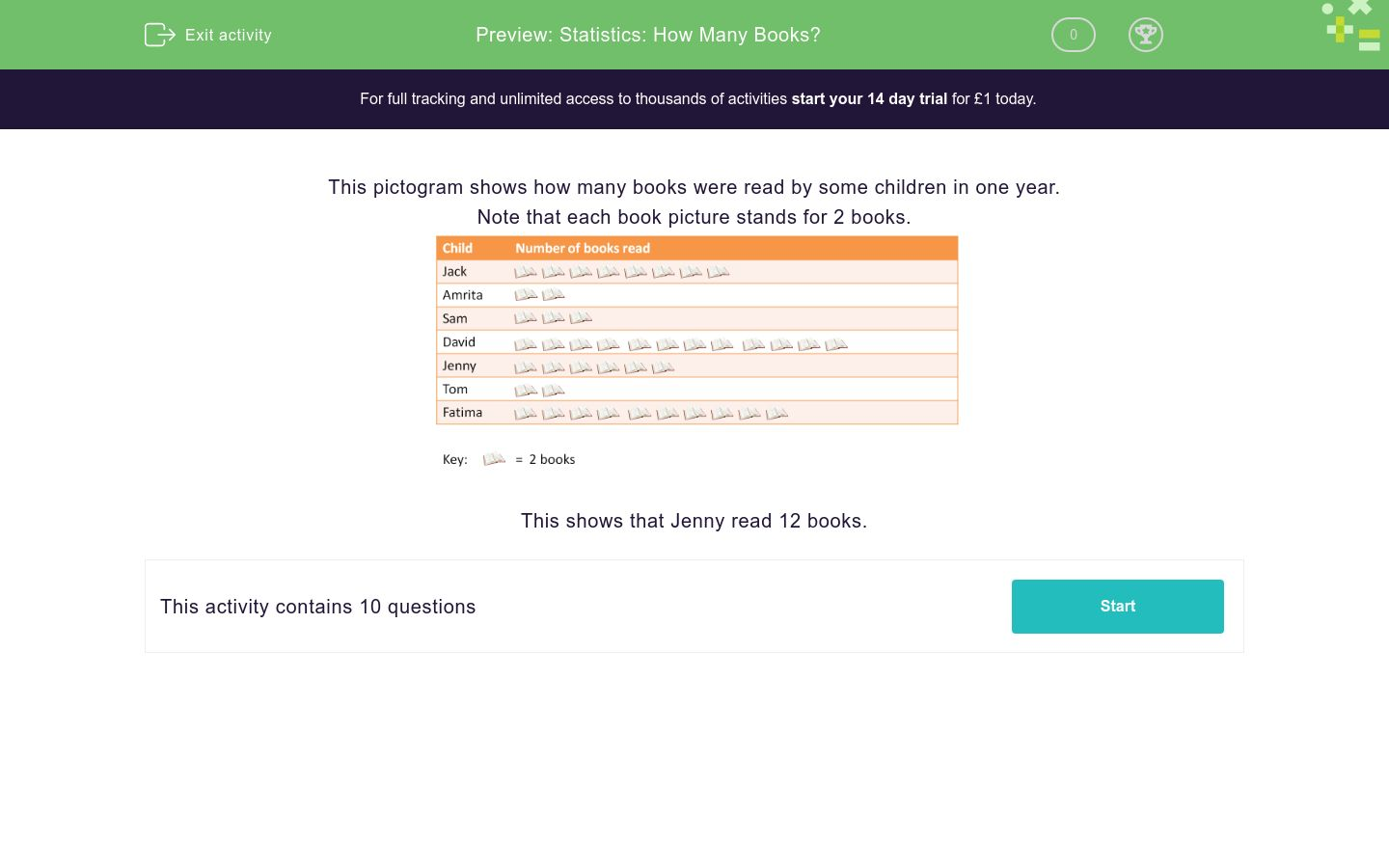# Statistics: How Many Books?

In this worksheet, students read information from a pictogram and answer questions on it.Key stage:  KS 2

Curriculum topic:   Statistics

Curriculum subtopic:   Solve Statistic Problems

Difficulty level:### QUESTION 1 of 10

This pictogram shows how many books were read by some children in one year.

Note that each book picture stands for 2 books.This shows that Jenny read 12 books.

Use the pictogram to answer the question.

How many books did Jack read?Use the pictogram to answer the question.Use the pictogram to answer the question.

Who read the least number of books?Use the pictogram to answer the question.

Who read an odd number of books?Jack

Amrita

Sam

Nobody

Use the pictogram to answer the question.

Who read more than 7 books?Jack

David

Jenny

Fatima

Use the pictogram to answer the question.

Who read fewer than 5 books?Amrita

Sam

Jenny

Tom

Use the pictogram to answer the question.

How many books did Jack and Sam read altogether?Use the pictogram to answer the question.

How many more books did Sam read than Amrita?Use the pictogram to answer the question.

Which person read as many books as Amrita, Tom and Jenny put together?Use the pictogram to answer the question.

How many books were read altogether?• Question 1

Use the pictogram to answer the question.

How many books did Jack read?16
EDDIE SAYS
Jack has 8 books in the pictogram and each book represents 2, so 8 x 2 = 16. Jack read 16 books.
• Question 2

Use the pictogram to answer the question.David
EDDIE SAYS
David has 12 books in the pictogram and each book represents 2, so 12 x 2 = 24. David read the most books.
• Question 3

Use the pictogram to answer the question.

Who read the least number of books?Amrita
Tom
EDDIE SAYS
Both Amrita and Tom only read 4 books each (2 x 2 = 4).
• Question 4

Use the pictogram to answer the question.

Who read an odd number of books?Nobody
EDDIE SAYS
• Question 5

Use the pictogram to answer the question.

Who read more than 7 books?Jack
David
Jenny
Fatima
EDDIE SAYS
Jack, David, Jenny and Fatima read more than 7 books each.
• Question 6

Use the pictogram to answer the question.

Who read fewer than 5 books?Amrita
Tom
EDDIE SAYS
Amrita and Tom only read 4 books each.
• Question 7

Use the pictogram to answer the question.

How many books did Jack and Sam read altogether?22
EDDIE SAYS
Jack read 16 books and Sam read 6 books. Altogether they read 22 books. 16 + 6 = 22.
• Question 8

Use the pictogram to answer the question.

How many more books did Sam read than Amrita?2
EDDIE SAYS
Sam read 6 books and Amrita read 4. Sam read 2 more books than Amrita. 6 - 4 = 2.
• Question 9

Use the pictogram to answer the question.

Which person read as many books as Amrita, Tom and Jenny put together?Fatima
EDDIE SAYS
Amrita, Tom and Jenny read 20 books altogether. 4 + 4 + 12 = 20 Fatima read 20 books, as many as them altogether.
• Question 10

Use the pictogram to answer the question.

How many books were read altogether?86
EDDIE SAYS
Add all of the books together to get the total: 8 + 2 + 3 + 12 + 6 + 2 + 10 = 43 43 x 2 = 86 books
---- OR ----